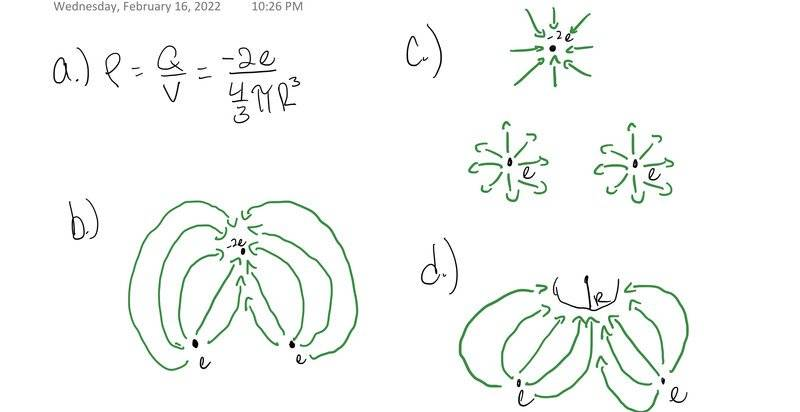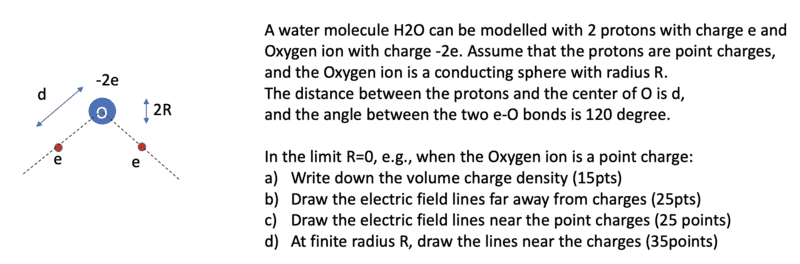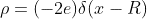# Electric field lines of H2O molecule

guyvsdcsniper
Homework Statement:
In the limit R=0, e.g., when the Oxygen ion is a point charge:
a) Write down the volume charge density (15pts)
b) Draw the electric field lines far away from charges (25pts)
c) Draw the electric field lines near the point charges (25 points)
d) At finite radius R, draw the lines near the charges
Relevant Equations:
p=q/v
I wanted to post my work so far to see if I am on the right path toward the correct answer so far.

I have attached a ss of the actual problem and my work in the attachments#### Attachments

Homework Helper
Gold Member
Part (a) does not look correct. Remember, when you integrate the volume charge density over all space, you should get zero. Also, you are told to model the oxygen as a conducting sphere of radius R. Is the charge distributed uniformly over the volume of the sphere?

Part (c) needs some fixing. You must have twice as many lines going into the sphere as coming out of either one of the charges. Do you see why? Parts (b) and (d) look OK.

•guyvsdcsniper
guyvsdcsniper
Part (a) does not look correct. Remember, when you integrate the volume charge density over all space, you should get zero. Also, you are told to model the oxygen as a conducting sphere of radius R. Is the charge distributed uniformly over the volume of the sphere?

Part (c) needs some fixing. You must have twice as many lines going into the sphere as coming out of either one of the charges. Do you see why? Parts (b) and (d) look OK.
for part (a) it should be zero because I followed the diract delta function, I should get a spike at the center and zero all over space correct?

part (c), Is this due to having 2 electrons?

Homework Helper
Gold Member
for part (a) it should be zero because I followed the diract delta function, I should get a spike at the center and zero all over space correct?

part (c), Is this due to having 2 electrons?
You need Dirac deltas for all three charges. When you put charge -2e on a conductor of radius R, does the charge go to the center?

For part (c) draw Gaussian surfaces around each charge. The number of electric field lines coming out or going in must be proportional to the enclosed charge.

guyvsdcsniper
You need Dirac deltas for all three charges. When you put charge -2e on a conductor of radius R, does the charge go to the center?

For part (c) draw Gaussian surfaces around each charge. The number of electric field lines coming out or going in must be proportional to the enclosed charge.
No it wouldn't go to the center. It would spread evenly on the surface. So for the electron it should be,?
I am not sure about the protons though? I don't know the center of the protons.